# Can a Machine Learn a Power Spectrum Estimator?

I continue with my foray into machine learning (ML) by considering whether we can use widely available ML tools to create a machine that can output accurate power spectrum estimates. Previously we considered the perhaps simpler problem of learning the Fourier transform. See here and here.

Along the way I’ll expose my ignorance of the intricacies of machine learning and my apparent inability to find the correct hyperparameter settings for any problem I look at. But, that’s where you come in, dear reader. Let me know what to do!

### The Power Spectrum

First, what is the power spectrum? It is often called the power spectral density (PSD), and it is the spectral density of time-averaged energy (power). I define it in detail in the post on a closely related function called the spectral correlation function. I also consider estimators and the nature of an important power-related function called the periodogram in another post.

If we have a random persistent power signal$x(t)$, we denote its power spectrum by the symbol$\displaystyle S_x^0(f)$ or more conventionally by simply$\displaystyle S_x(f)$. It has units of Watts/Hz. So when we want to find the power, in Watts, that the signal possesses on the average in some frequency band$\displaystyle [f_1, f_2]$, we can integrate the density:$\displaystyle P([f_1, f_2]) = \int_{f_1}^{f_2} S_x^0(f) \, df \hfill (1)$

If we want the total power for the signal, we integrate over all possible frequency values,$\displaystyle P_x = \int_{-\infty}^\infty S_x^0(f) \, df \hfill (2)$

which is consistent with the Wiener-Khinchin relation between the power spectrum and the autocorrelation function,$\displaystyle R_x^0(\tau) = \int_{-\infty}^\infty S_x^0(f) e^{i 2 \pi f \tau} \, df \hfill (3)$

because when$\tau = 0$, the autocorrelation$R_x^0(0)$ is just the power of$x(t)$ so that$\displaystyle R_x^0(0) = P_x = \int_{-\infty}^\infty S_x^0(f) \, df. \hfill (4)$

### PSD Estimators and our Machine-Learning Objective

One reason I care about getting a machine to learn how to estimate the PSD is that I’ve encountered multiple machine learners who profess the desire to input raw data samples and have the machine perform automatic modulation recognition (see the Challenge post). But then they don’t actually input just the data samples, they also add the spectrogram as an input. Why not just let the machine figure out if it needs something like the spectrogram? Maybe it needs it, maybe it doesn’t. Well, they don’t come out and say “I’ve tried to get my machine to learn the spectrogram, but I can’t do it.” So I wonder if it can be done. Instead of the spectrogram, which is essentially a temporal sequence of PSD estimates, I’ll try the simpler (or so I think [OSIT]) single PSD estimate.

Estimators of the power spectrum include many parametric methods, which assume one or another explicit mathematical model for the signal and/or noise (such models have “parameters”), and non-parametric methods, which do not impose a model on the signal and noise. The two main non-parametric estimators are the time-smoothed periodogram (what I call the TSM) and the frequency-smoothed periodogram (FSM). In this post, we’ll see if we can get a machine to learn the time-smoothed periodogram method for an input consisting of a relatively large number of samples, such as$8192$, and an output PSD with a relatively small number of frequency points, such as$128$.

What I mean by “learn the TSM” is not that the machine will have weights that somehow we can look at and say: Ah, it is doing the mathematical operations that make up the TSM, namely a set of Fourier transforms, a magnitude-squaring operation, and an averaging operation, in that order. Instead, I just mean that the machine settles on an operation on the data that gives a good approximation to TSM-created PSDs. Maybe the learned neural network will be faster or even better than the TSM and/or FSM.

### An Early Attempt Using Short Inputs and the FSM

I spent a lot of time on a simpler (OSIT) version of the problem. Each complex-valued input had a length of$128$ samples and I used the FSM to estimate the PSD, which is a real-valued vector of length$128$. Taking the various machine-learning papers I’ve read to heart, I embraced a systematic trial-and-error approach to setting the hyperparameters. (I don’t think I’ve read a machine-learning paper that didn’t admit to using a trial-and-error approach to finding a good set of hyperparameters.) My machine-learning tool is MATLAB’s Deep Learning toolbox. The trial-and-error work was tedious, and I eventually quit before I tried all possible combinations of parameters. Here is the log of my efforts: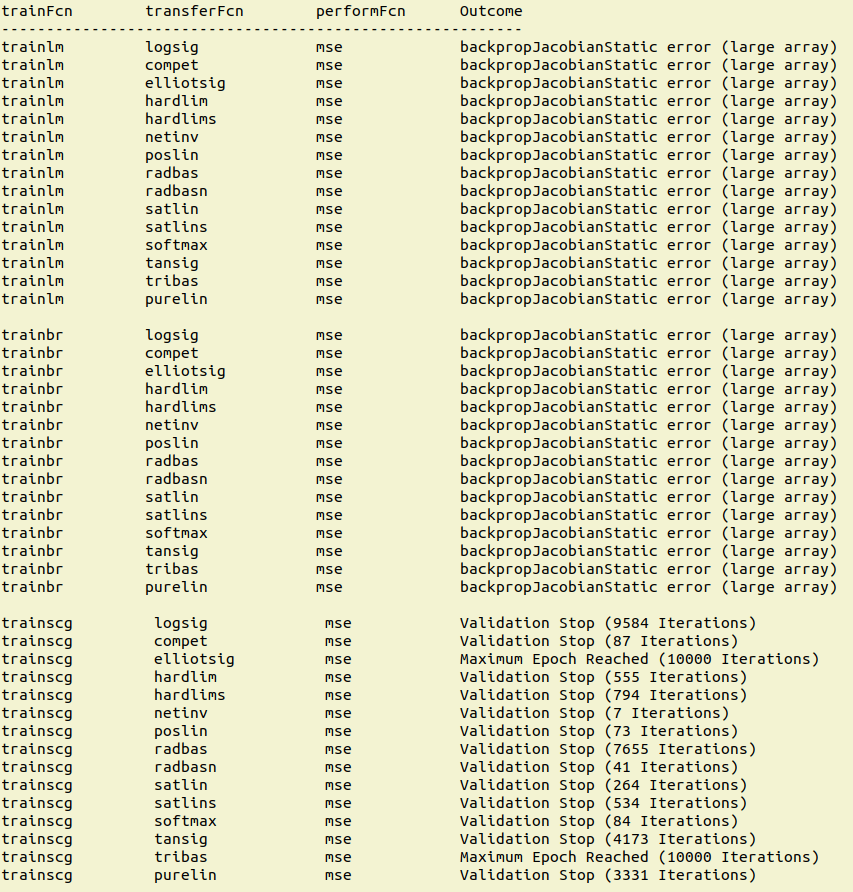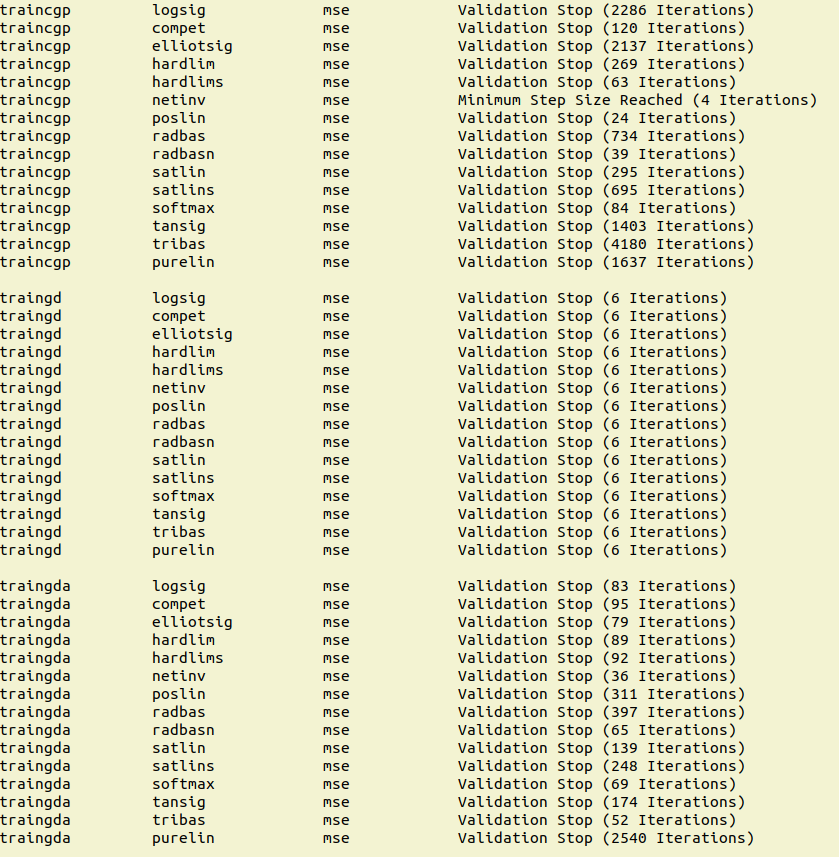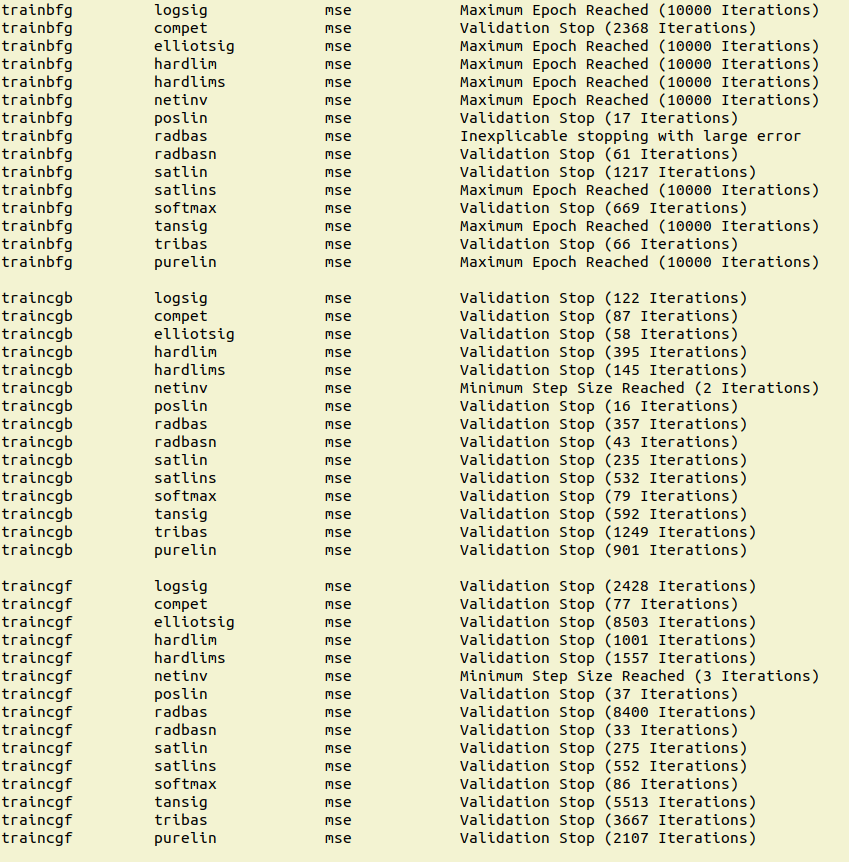Then I moved on to the problem of main interest in the present post, which uses a longer input ($8192$ samples) and the TSM to estimate the PSD, where the TSM block length is$128$ frequency points. So let’s talk about that next.

### The Training Set

To train the machine, I created a variety of signal types, including WGN, sine waves, BPSK, QPSK, 2FSK, 4FSK, DSSS BPSK, and OFDM. There are$400$ instances of each of those types for a total data set size of$3600$ waveforms. Each waveform has$8192$ complex-valued samples and each corresponding PSD estimate has$128$ real-valued samples (the TSM block size is$128$.). The inputs are reformed from complex-valued vectors having$8192$ samples into real-valued vectors having$16384$ samples by concatenating the real and imaginary parts.

The message sequences of the communication signals are statistically independent from waveform to waveform, as is added white Gaussian noise. The relative amount of noise and signal is varied to achieve a variety of SNRs.

The input waveforms and output PSD estimates can be downloaded from the CSP Blog here.

To fix the ideas, let’s plot some of the inputs and the corresponding outputs.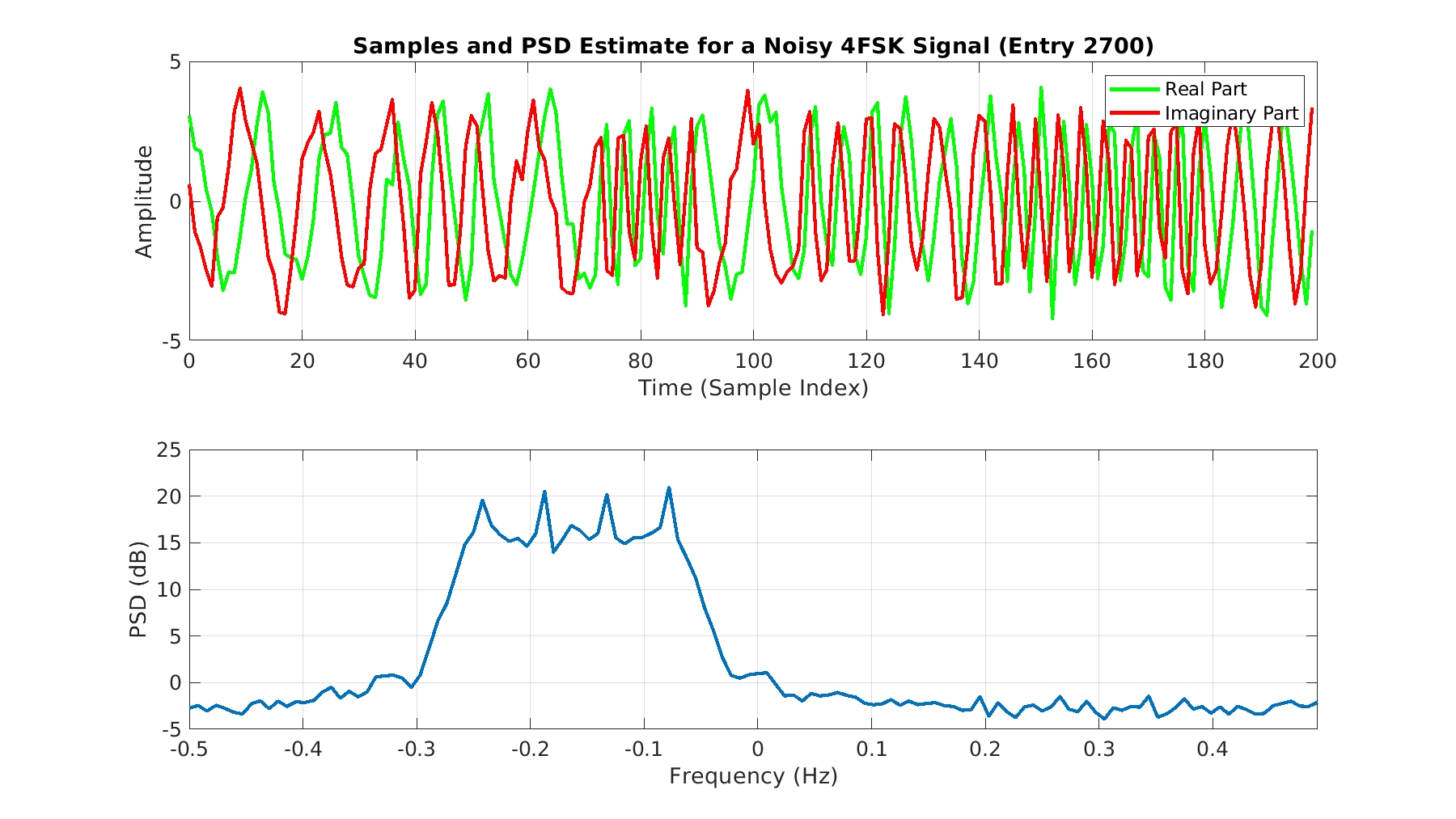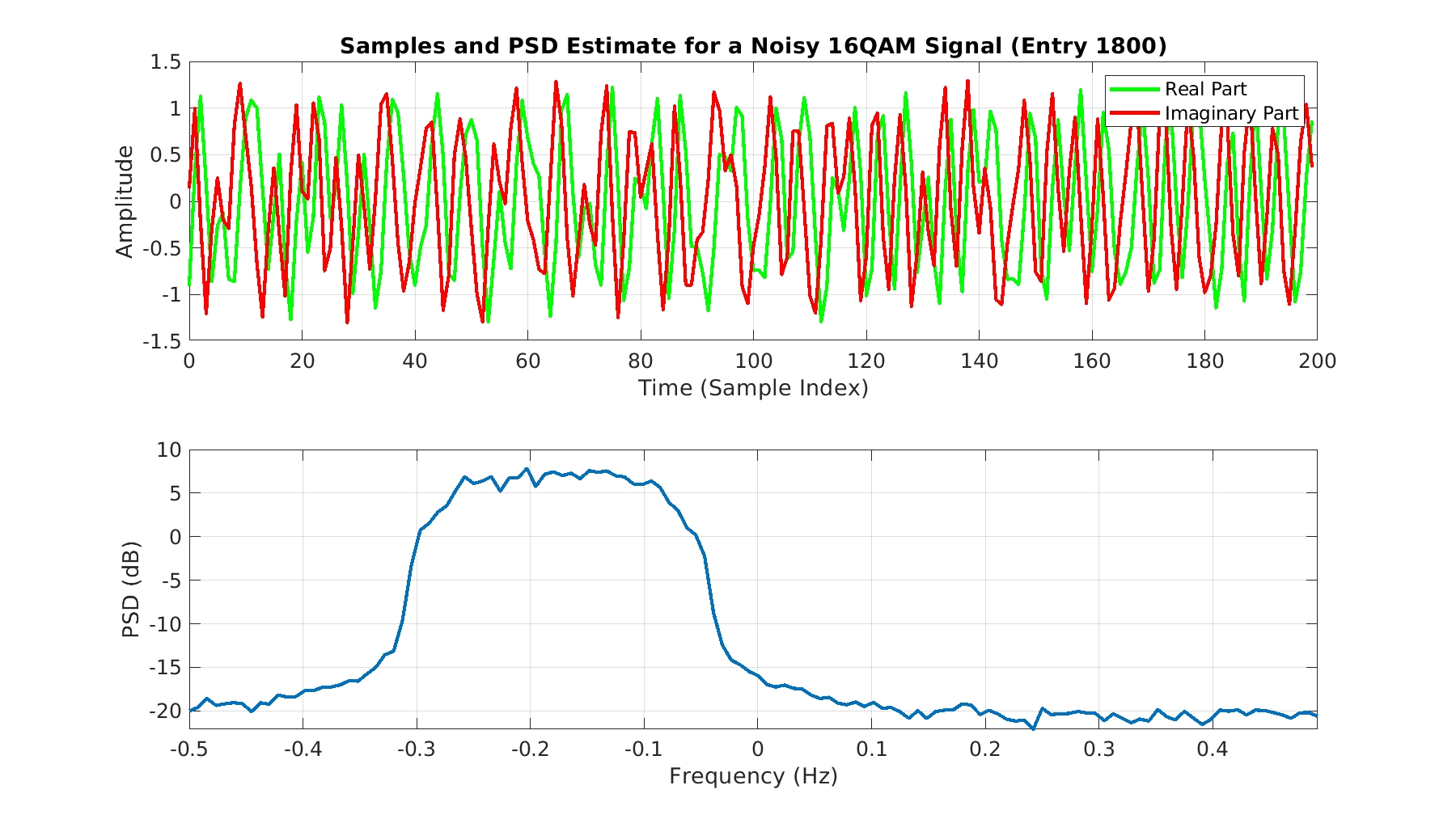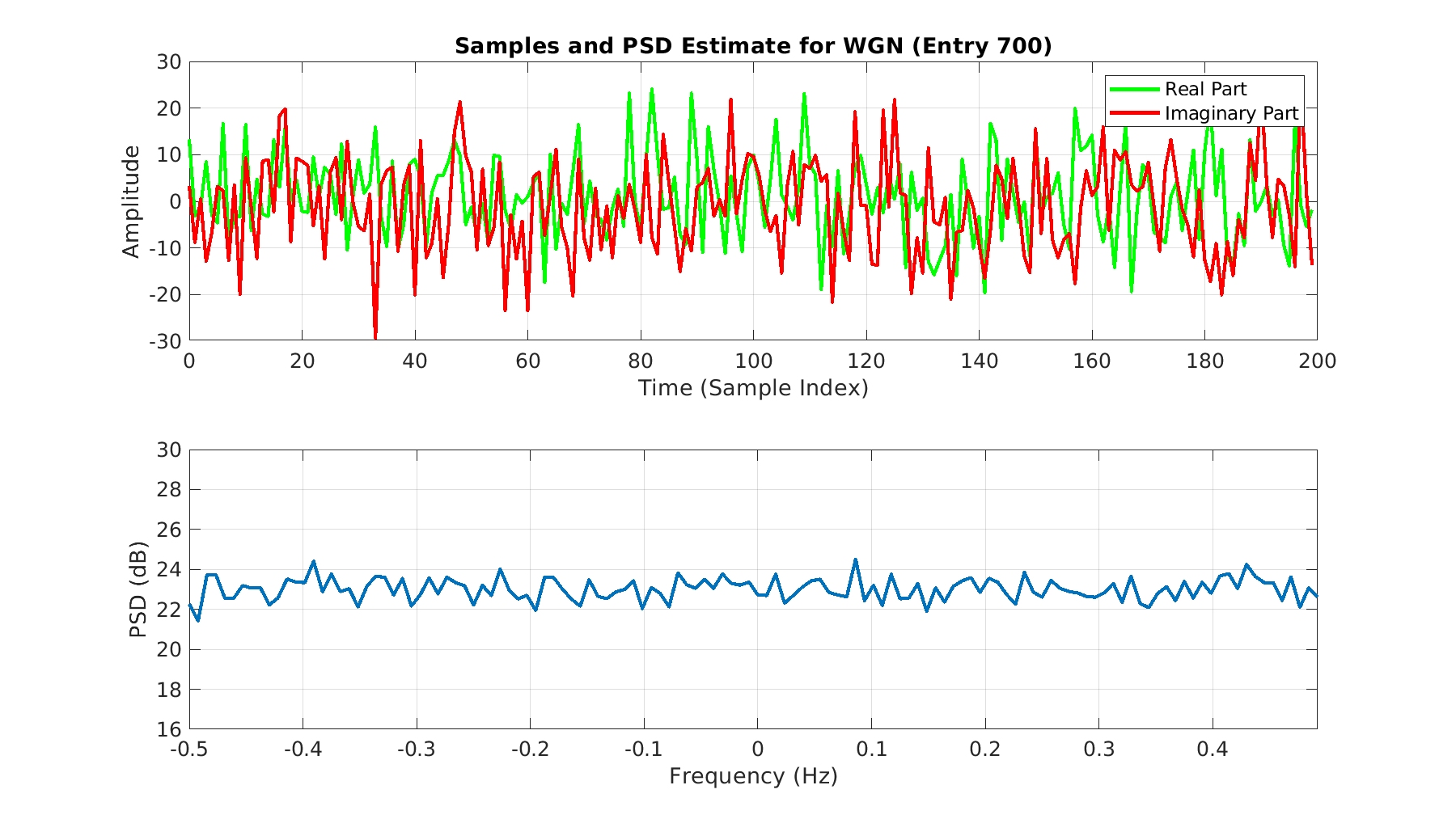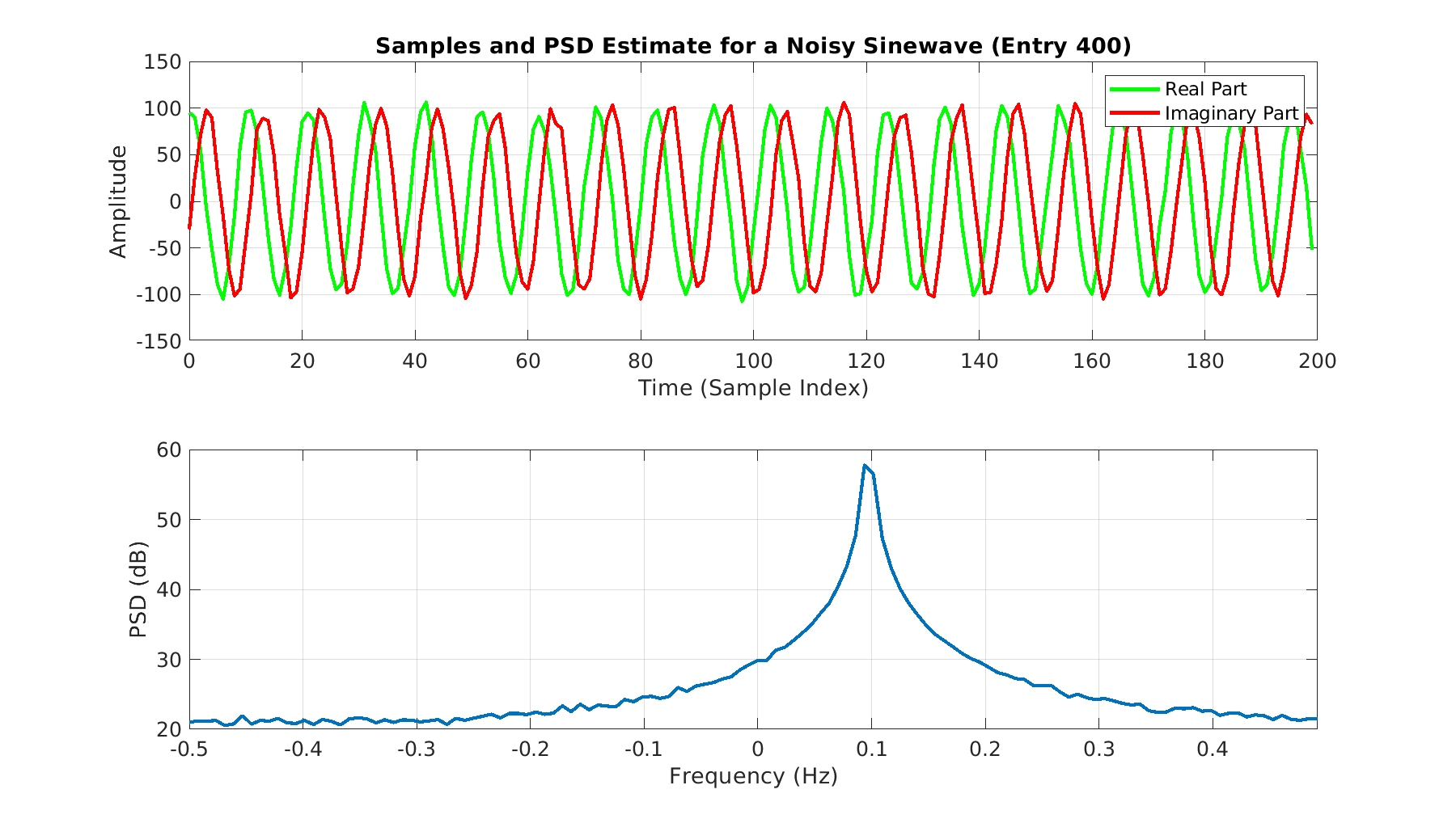### The Training Code

The basic MATLAB function I used to do the trial-and-error hyperparameter selection can be found here.

### Some Training Results

#### Full Data Set

I’ve not had the patience to redo for the long-duration inputs what I did for the shorter ones (see above). Here are some results:#### Noise-Only Data Set

The next experiment again uses long inputs, but restricts their content to white Gaussian noise. So all the signals that we use to train the machine are WGN, and therefore the theoretical PSDs are simply constant functions of frequency. The TSM estimates in the training and testing data sets are not perfectly constant, however, but are quite flat (see above). Here the machine has an easier task (OSIT) since the height of the correct PSD output is simply the variance of the input. It is nearly the simplest nonlinear transformation of the input that is possible (Can a Machine Learn the Square of an Input would consider the simplest nonlinear transformation, I suppose).

Here is the performance curve for the case of a training function of SCG, a transfer function of logsig, and the MSE performance criterion: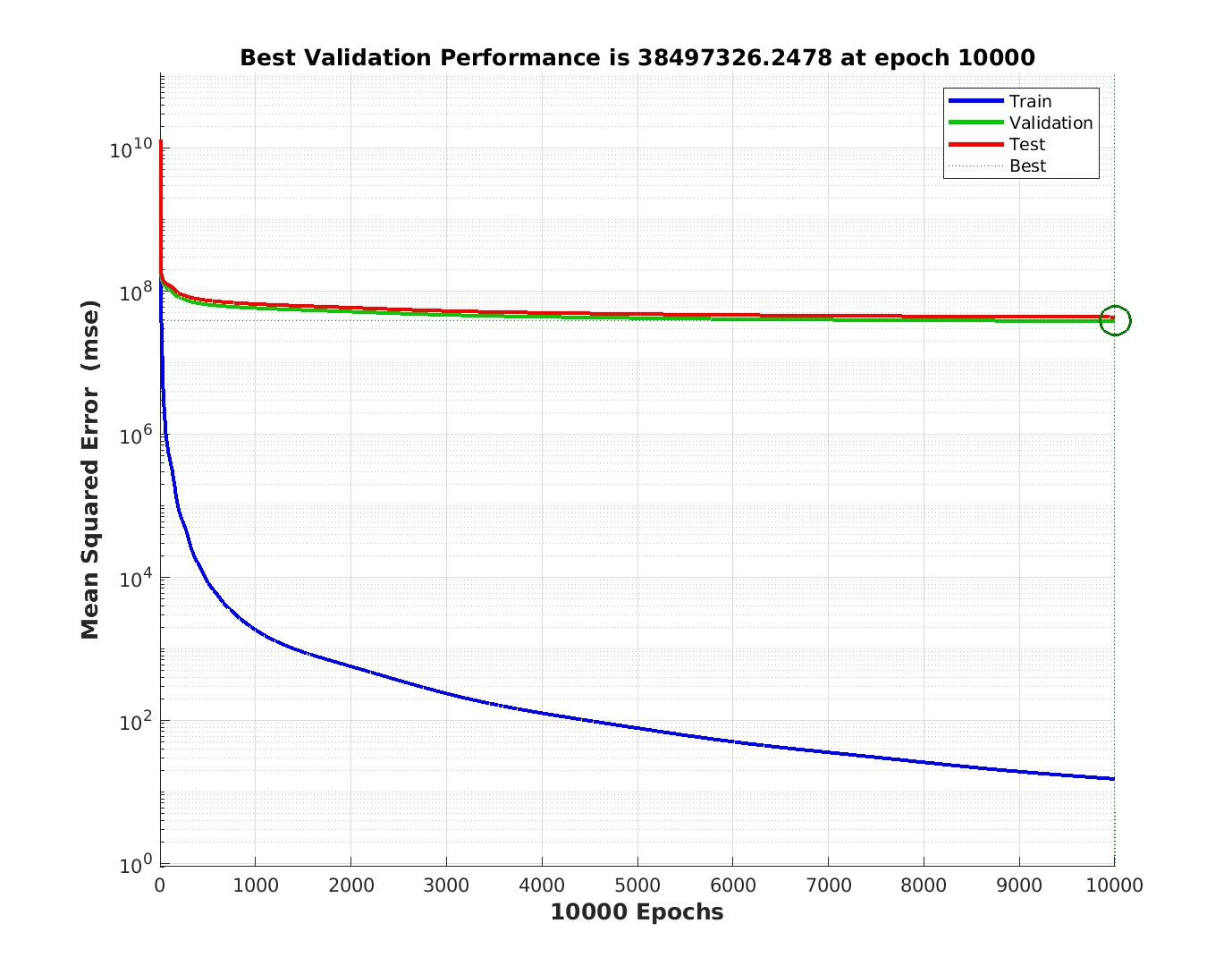In this instance, the training stopped after the maximum number of iterations were reached, but the performance on the testing data set is nowhere near that for the training set (MATLAB itself divides the provided data set into training and testing subsets by default).

I also attempted training with both the noise-only and the full data set for a performance criterion that I believe is normalized MSE, rather than MSE, to avoid having the machine minimize the error by attempting to match the most powerful inputs and just living with the resulting errors for the low-power inputs. These were not successful either. The modified training code for NMSE can be downloaded here.

### Conclusions

My first conclusion is that I’ve reaffirmed I don’t know what I’m doing in Machine Learning. That is probably not a surprise to regular readers. The second is that even with a lot of trial-and-error I cannot get good results for training a machine to produce a PSD estimate, even when the universe of inputs is constrained to white Gaussian noise so that the PSD is always a constant function.

Does anyone have any suggestions for adjusting the hyperparameters to allow the machine to learn how to estimate a PSD?

## 4 thoughts on “Can a Machine Learn a Power Spectrum Estimator?”

1.Ilya says:

Hi!
As far as i understand you are using ‘logsig’ activation that returns [0, 1] as an output layer. Also mapminmax modifies it to [-1, 1] range. But PSD plots show values ~10 and more. If you do regression with mse as a loss function – that could be a problem. I think it worths to try mapminmax for input only and use unconstrained activation (e.g. ReLu).
Also, it feels like this task is better suited for 1D convolutional networks (because translating an input doesn’t change PSD, isn’t it?).

Best Regards,
Ilya
P.S. Thank you for your blog!

•Chad Spooner says:

Thanks very much for reading the blog and offering your ML insights Ilya!

You are correct that the PSD of a signal does not change with time translation.

The results I showed for the longer-input case do correspond only to the logsig activation function. I ran out of patience unfortunately. Also in that longer-input case, I did some logsig runs with the mapminmax and some without.

But in the long table in the post, for the shorter-input case using the FSM, I used a variety of different activation functions. None were successful. Do you have any comment on that result?

2.Christopher Moore says:

Hey this is great. I’m going to do something related for a school project at UVA! Super excited will check back in! good work!

•Chad Spooner says:

Christopher:

Thanks for stopping by the CSP Blog. I’m hoping you can eventually set me straight on my attempt to use ML to create a PSD estimator. Looking forward to hearing from you.